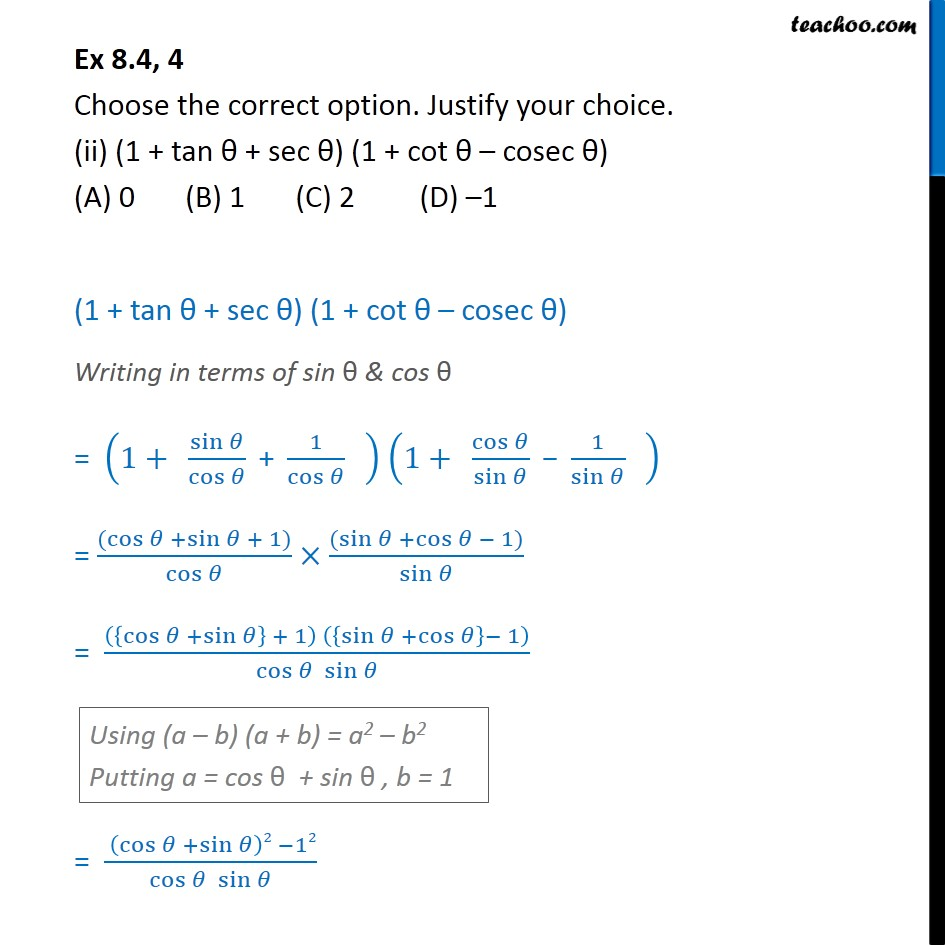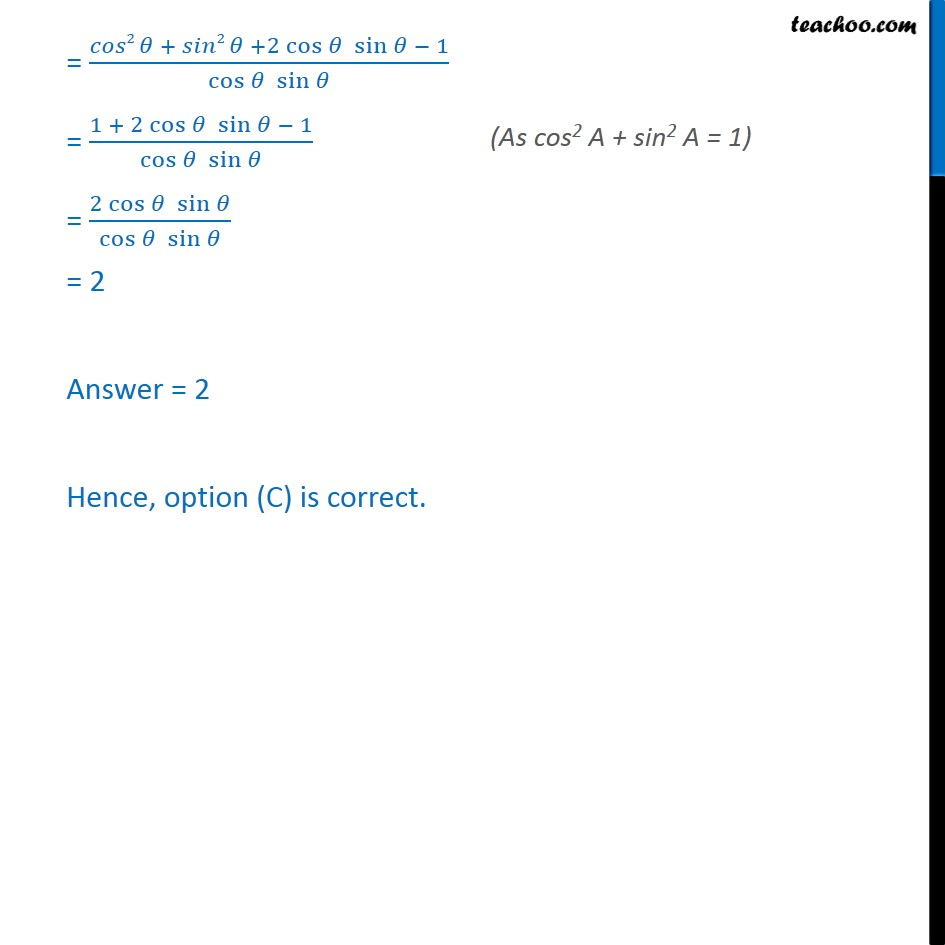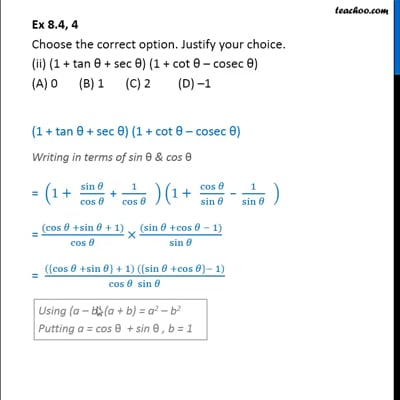Ex 8.4

Chapter 8 Class 10 Introduction to Trignometry
Serial order wiseThis video is only available for Teachoo black users

Maths Crash Course - Live lectures + all videos + Real time Doubt solving!

### Transcript

Ex 8.4, 4 Choose the correct option. Justify your choice. (ii) (1 + tan θ + sec θ) (1 + cot θ – cosec θ) (A) 0 (B) 1 (C) 2 (D) –1 (1 + tan θ + sec θ) (1 + cot θ – cosec θ) Writing in terms of sin θ & cos θ = (1+" " sin⁡𝜃/cos⁡𝜃 " + " 1/cos⁡𝜃 " " ) (1+" " cos⁡𝜃/sin⁡𝜃 " − " 1/sin⁡𝜃 " " ) = ((cos⁡𝜃 +sin⁡𝜃 + 1))/cos⁡𝜃 × ((sin⁡𝜃 +cos⁡𝜃 − 1))/sin⁡𝜃 = (({cos⁡𝜃 +sin⁡𝜃 } + 1) ({sin⁡𝜃 +cos⁡𝜃 }− 1))/(cos⁡𝜃 sin⁡𝜃 ) = ( (cos⁡𝜃 +sin⁡𝜃 )2 −12)/(cos⁡𝜃 sin⁡𝜃 ) = (𝑐𝑜𝑠2 𝜃 + 𝑠𝑖𝑛2 𝜃 +2 cos⁡𝜃 sin⁡𝜃 − 1)/(cos⁡𝜃 sin⁡𝜃 ) = (1 + 2 cos⁡𝜃 sin⁡𝜃 − 1)/(cos⁡𝜃 sin⁡𝜃 ) = (2 cos⁡𝜃 sin⁡𝜃)/(cos⁡𝜃 sin⁡𝜃 ) = 2 Answer = 2 Hence, option (B) is correct.